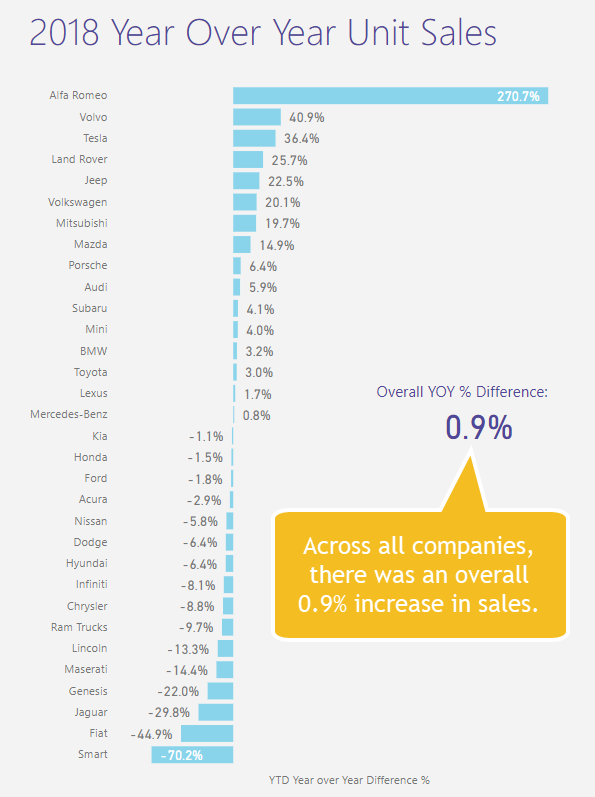# The Antares Alien

## Power BI   |  Game Development

Search
•Brent Jones

# Does the 80/20 Rule Appear in Car Sales? A Power BI AnalysisI always here about the 80/20 rule in theory but I've never actually gone out and tried to find it myself. So I decided to test it out with US car sales. Like my other blogs, I'll go through my findings of the data, and then afterwards I'll go over how I built the dashboard including the techniques and calculations I used.

### The Data

It's rather difficult finding good data sources without having to pay (who wants to pay, right?), but I did find one website with some relatively easy-to-access data at goodcarbadcar.net.

They provided quite an extensive list of automakers, model, and unit sales figures for this year and last year, which I simply transferred over to an excel spreadsheet. From that, I was able to build the following data dashboard:

### Analysis

Okay, now onto the question. From this data, I can ask two questions actually:

• Do 80% of the sales come from 20% of the countries in the US?

• Do 80% of the sales come from 20% of the companies in the US?

I ended up seeing more of an 80/30 rule in both cases...

For Countries:For Companies:### Tesla

I'm a big fan of Tesla (though I don't have one (yet!)), so I also wanted to do a bit of data viz showing off Tesla's stats. In the below image, we can see that they have about a 36% increase in year over year sales. This is in May of 2018, so I believe that number has grown much more with the rise in Model 3's.For comparison, including all companies there was only a 0.9% increase in year over year sales.### Dashboard Techniques

For this dashboard, I used two different custom visuals which you can get from the marketplace. The "Stacked Bar Chart By Akvelon" and the "Bar Chart with Relative Variance".

The "Stacked Bar Chart By Akvelon" allows you to select a region of the graph by clicking and dragging. I wanted this functionality so that users can visualize select a region of the graph that looks to be 20% or 30%, etc.. Although it looks like this feature doesn't work on the service, on the desktop it does provide an interesting and interactive way to view your data. Hopefully the developers can get this functionality to work in the service soon.The "Bar Chart with Relative Variance" provides an simple and unique way to show relative changes for your data. There is a bar chart which visualizes both values (i.e. before and after, year over year, etc.) and a thin variance bar chart on the right hand side which represents the % change for the respective category.### DAX Tips and Calculations

The prominent calculation I used in this project was a percentage of total represented by:

% of Total = Current Value / Total

Due to filters interacting with Dax calculations, it can be a bit tricky to get it right. The key is to use the Calculate( ) function in tandem with normal aggregation functions like SUM( ). For example, to calculate the percent of sales in the following image...I'm using two DAX measures. First, I create a normal SUM( ) measure for total sales:

Total Sales = Sum( 'Sales Data'[YTD Sales])

### Calculate( )

My next DAX measure, I'll reference the above measure, and use a Calculate( ) function as well:

Total Sales % =

var totalSales = CALCULATE([Total Sales], All('Sales Data'))

var selection = [Total Sales]

return

selection / totalSales

You can see that I'm referencing [Total Sales] twice. Once in the Calculate( ) function, and another for the selection variable. But what does this Calculate( ) function achieve for us? Calculate( ) allows us to 'rewrite' the filtering operations that Power BI does.

Basically, if you use Calculate( ), you can tell Power BI exactly how you would like to filter a calculation (similar to a SUMIF in Excel). I need to calculate the sum of all sales, regardless of any filters to use as my base. In my formula above, I'm using the All( ) function to achieve this. The All( ) function tells Power BI to ignore any filters that may be applied on the page and use the entire table for the calculation.

The complete function, CALCULATE([Total Sales], All('Sales Data')), is taking the instructions for the first measure, [Total Sales], and calculating it using ALL the records from the 'Sales Data' table - again, ignoring any filters that are applied on the page.

So I'll take that value from the Calculate( ) function and store it in a variable called totalSales. Finally, I'm telling DAX to RETURN a value resulting from the operation, selection / totalSales, which equals the total sales percentage.There are many other things you can accomplish using Calculate(), but this is the one I used for this project. I encourage you to explore more about this function, because it's quite powerful. I talk a little bit more about it in my other blog, Basic Power BI Dax Concepts.

I hope you found something of interest here! Let me know your thoughts in the comments and please share with your friends and coworkers!

Check out Supercharge Power BI by Matt Allington! Even if you think know DAX "just enough", this book will definitely help you like it did for me.

Support my Indie game!
Steam

Itch.Io

Support my Indie game!
Steam

Itch.Io